## Python Pandas for Data Science

##### In this part of Learning Python we Cover Pandas for Data Science in Python
Written by |30-Jul-2019 | 0 Comments | 854 Views

In this section, we will see how to implement pandas code in python. So first, we will see how to input the data in pandas.

Input The file:

Pandas support many types of the file type to load the data. A user can load CSV file, excel file, JSON file, SQL database, website URL which contains tabular data. We will see some of them below:

To load the CSV following is the code:

`import pandas as pd`
`df = pd.read_csv('titanic.csv')`
`df`

In the first line, I import pandas as pd to short the module name. Then we create a data frame in which I load the titanic data and then show that data frame. The output that will be as follows: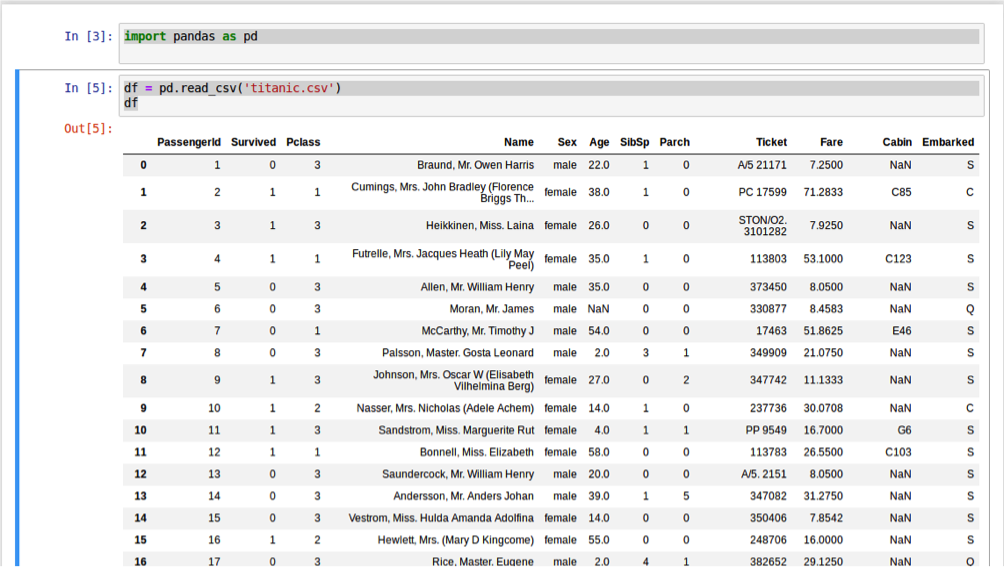Figure 1

It shows all the data that is in the CSV file in a proper table. It is also one of the reason of pandas popularity that its show the data in an appropriate formatted manner to understand more.

If a website contains HTML tabular data than it is straightforward with pandas to extract the data from that website directly. However, it is to be noted that pandas are not able to access the dynamic websites tabular data or websites that use JavaScript to load the tables. In such a scenario, other means of web scraping should be performed.

Suppose we have a website like W3School and we want to extract the tabular data out of it. The website looks like this:Figure 2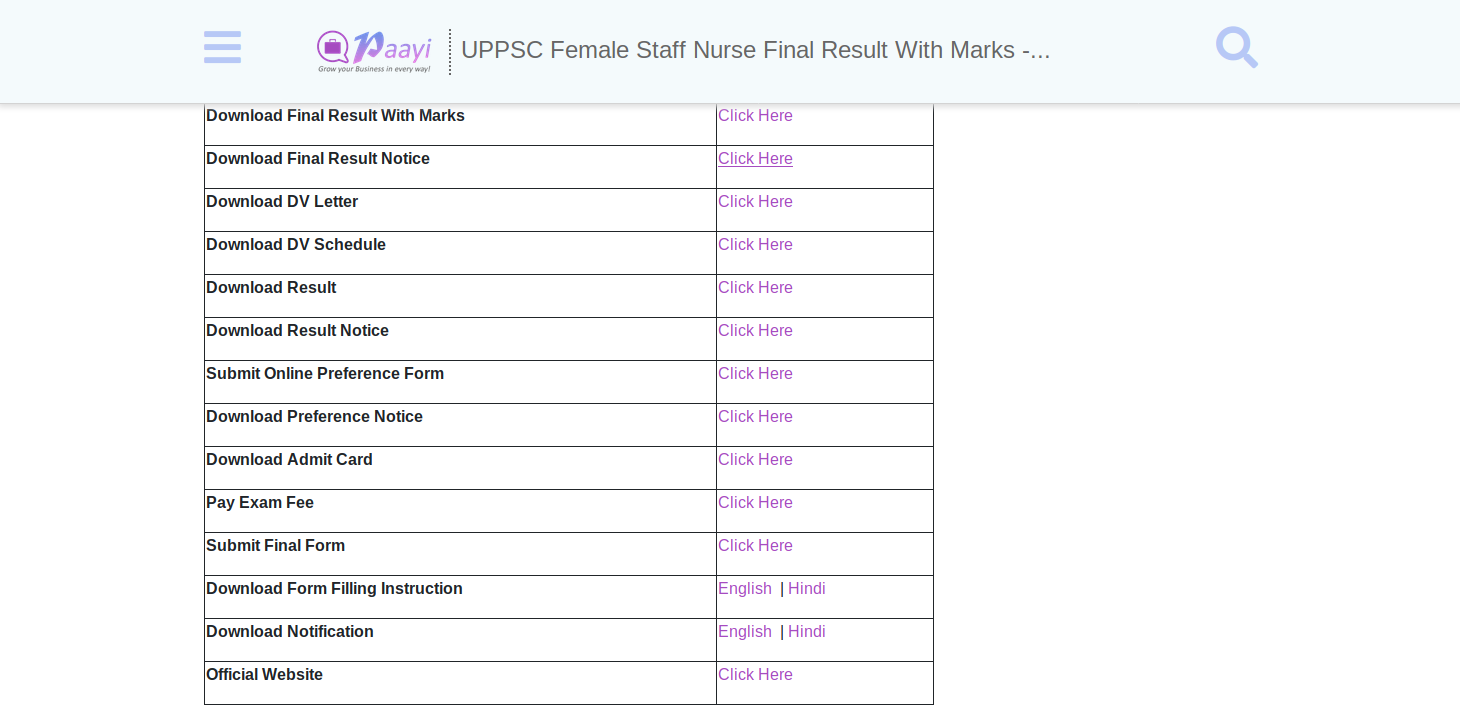Figure 3

Following code is executed to extract the data from these two tables:

`Import pandas as pd`
`df = pd.read_html('https://sarkariresult.paayi.com/uppsc-female-staff-nurse-final-result-with-marks-2019')`
`df`

However, if we execute the above code following output is generated:

It returns the list which contains all the tables that are present on the web page. So we have to slice the list and get an only 1st table so our code will look like this:

`Import pandas as pd`
`df=pd.read_html('`https://sarkariresult.paayi.com/uppsc-female-staff-nurse-final-result-with-marks-2019`')`
`df`

Moreover, the output can be seen as follows: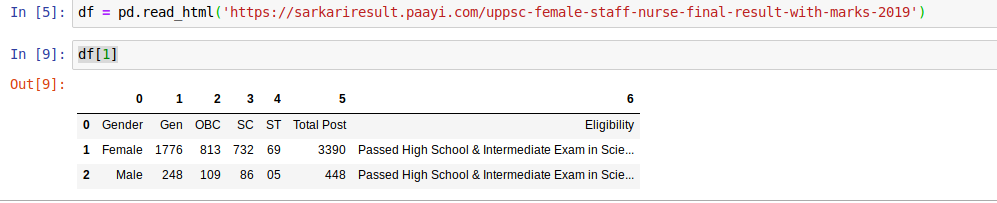Figure 4

Similarly, the second table output looks like this: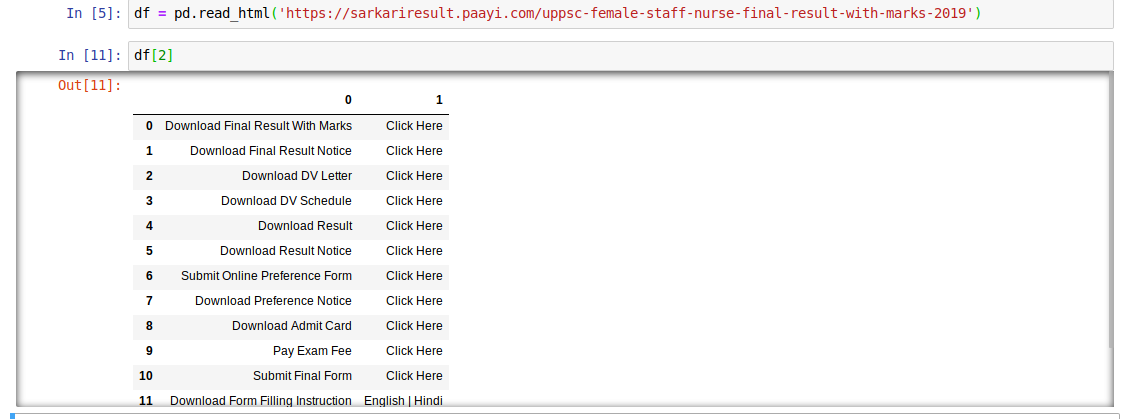Figure 5

Similarly, we can import other files like JSON SQL, etc. in pandas. Even a text file in which data is separated by some value can be imported in pandas.

The head function provides the number of rows from starting. By default, it gives only five rows, but we can give the parameter as much as we want. Similarly, the tail function provides the row from downward. Following is the code of implementation:

`import pandas as pd`
`df = pd.read_csv('titanic.csv')`
`df.head(10)`

The output as follows:Figure 6

The index starts from 0 to 9

Now we will see the tail function:

`import pandas as pd`
`df = pd.read_csv('titanic.csv')`
`df.tail(10)`

The output as follows: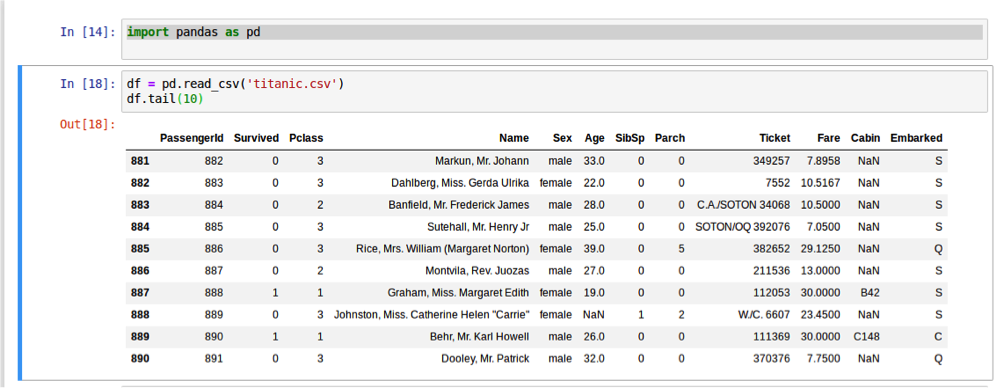Figure 7

Now we can see the index is from 881 to the last index. This how we can see that if data is loaded or not.

Indexing:

For indexing in pandas, there is a function which is iloc. It takes two parameters of rows and column. The rows and column parameter can further be slices by a colon.

Now we will see getting the data in different scenarios:

Getting 10 to 15 rows with all column:

`import pandas as pd`
`df = pd.read_csv('titanic.csv')`
`df.iloc[10:15 , ]`

In the above we can see iloc take two parameters 1st parameter is from 10 to 15 and second parameter after the coma is empty which means we want all of the columns the output that is generated is as follows: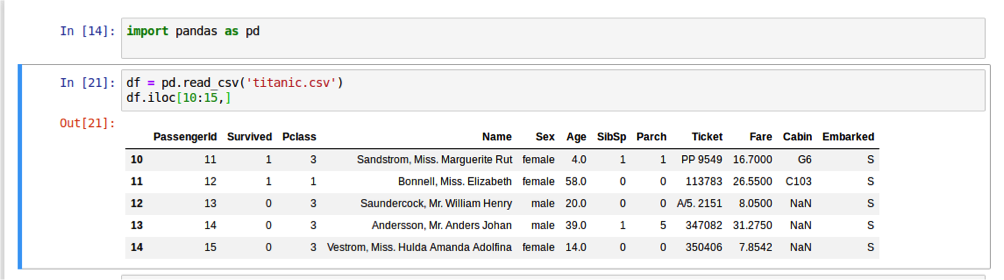Figure 8

### Getting Name and Sex of the first ten rows:

To get only name and sex from the table of only the first ten rows, the slicing will be as flows:

`import pandas as pd`
`df = pd.read_csv('titanic.csv')`
`df.iloc[:10,3:5]`

The output that is generated as a result is as follows: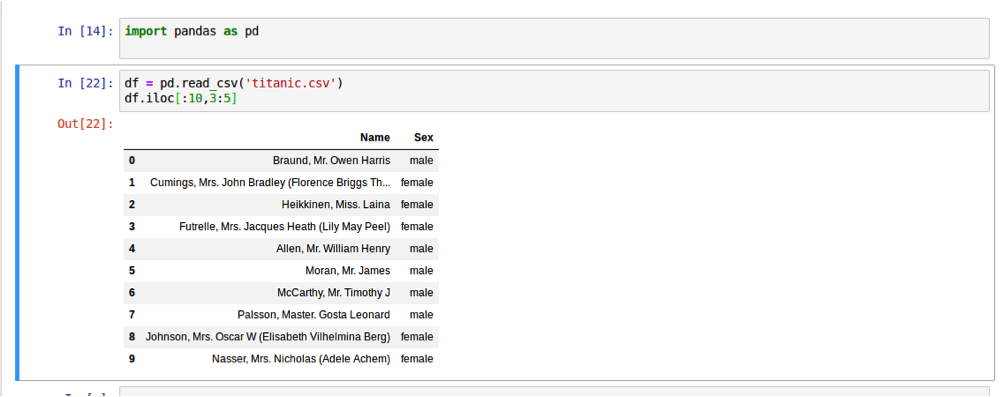Figure 9

Getting The column:

We can also get the columns by the header value. For multiple values, we have to give the list of columns as a parameter. The code is as follows:

`import pandas as pd`
`df = pd.read_csv('titanic.csv')`
`df['Name'][1:10]`

The output is as follows:

 1    Cumings, Mrs. John Bradley (Florence Briggs Th... 2                               Heikkinen, Miss. Laina 3         Futrelle, Mrs. Jacques Heath (Lily May Peel) 4                             Allen, Mr. William Henry 5                                     Moran, Mr. James 6                              McCarthy, Mr. Timothy J 7                       Palsson, Master. Gosta Leonard 8    Johnson, Mrs. Oscar W (Elisabeth Vilhelmina Berg) 9                  Nasser, Mrs. Nicholas (Adele Achem) Name: Name, dtype: object

Also, for multiple columns following modification has to be made:

`import pandas as pd`
`df = pd.read_csv('titanic.csv')`
`df[['Name','Sex']][1:10]`

The output is as follows:

 1    Cumings, Mrs. John Bradley (Florence Briggs Th..., Female 2                               Heikkinen, Miss. Laina, Female 3         Futrelle, Mrs. Jacques Heath (Lily May Peel), Female 4                             Allen, Mr. William Henry, Male 5                                     Moran, Mr. James, Male 6                              McCarthy, Mr. Timothy J, Male 7                       Palsson, Master. Gosta Leonard, Male 8    Johnson, Mrs. Oscar W (Elisabeth Vilhelmina Berg), Female 9                  Nasser, Mrs. Nicholas (Adele Achem), Female Name: Name, Sex dtype: object

Rename the Column Name:

Sometimes it is necessary to change the column name. It is also part of data cleaning. Sometimes the header name is long that it is impossible to memorize the name and write the spelling right. So, column rename took the dictionary of the existing name as the key and new name as its value. This function is performed by the rename function. The existing titanic dataset has the column Sex, and I want to rename it as a gender. So, to do so following is the method:

`import pandas as pd`
`df = pd.read_csv('titanic.csv')`
`df = df.rename(columns={'Sex':'Gender'})`
`df.columns`

Now if we call the df.columns following output will be generated:

 Index(['PassengerId', 'Survived', 'Pclass', 'Name', 'Gender', 'Age', 'SibSp',        'Parch', 'Ticket', 'Fare', 'Cabin', 'Embarked'],       dtype='object')

As we can see that Sex is now replaced by gender.

Fetching the Data according to conditions:

In this heading, we will see how we can extract the desired information only. For that purpose, we have to apply conditional operators like equals to, higher than, less than, not equals to, etc.

Let’s take an example of the Titanic dataset. We have to extract all the rows where the Sex is male. For that, we have to apply condition as follows:

`import pandas as pd`
`df = pd.read_csv('titanic.csv')`
`df = df[(df['Sex']=='male']`
`df.head(10)`

The output is as follows: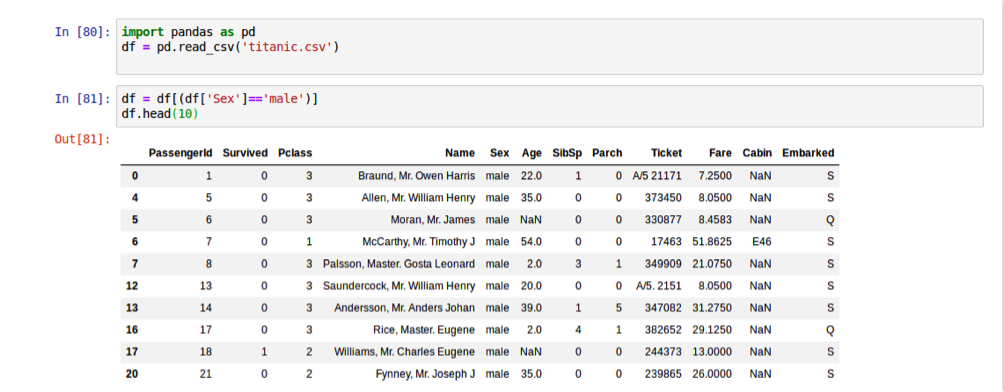Figure 10

The output that is generated is given in the above figure. We can see all the data is that which contains the male as sex.

We can further extend the conditions by applying an operation in it. Now we will extract all the data in which the sex is male, and the person survived that incident.

Following is the code to implement such a scenario:

`import pandas as pd`
`df = pd.read_csv('titanic.csv')`
`df = df[(df['Sex']=='male') & df['Survived']==1]`
`df.head(10)`

Now the output that is generated as follows: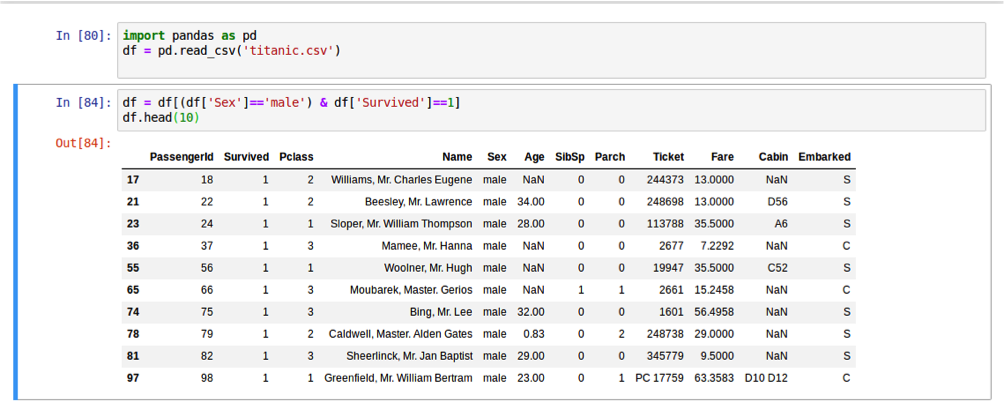Figure 11

Now the question arises why we have to do this? The answer to the question is if there is a problem to count how many males survived in a titanic incident so, after all that filtering, we then can calculate the number of rows and by which we can answer the question that this percentage of man was survived in a titanic incident.

IF Conditions:

In machine learning, the algorithm would not accept the element that is string character, so we have to convert it into a number before feeding into an algorithm. Suppose we have to convert the male into 1 and female as 0 we have to apply if condition and to do so following steps are involved:

`import pandas as pd`
`df = pd.read_csv('titanic.csv')`
`df['Sex'] = df['Sex'].apply(lambda x:1 if x=='male' else 0)`
`df.head(10)`

The output that is generated as follows: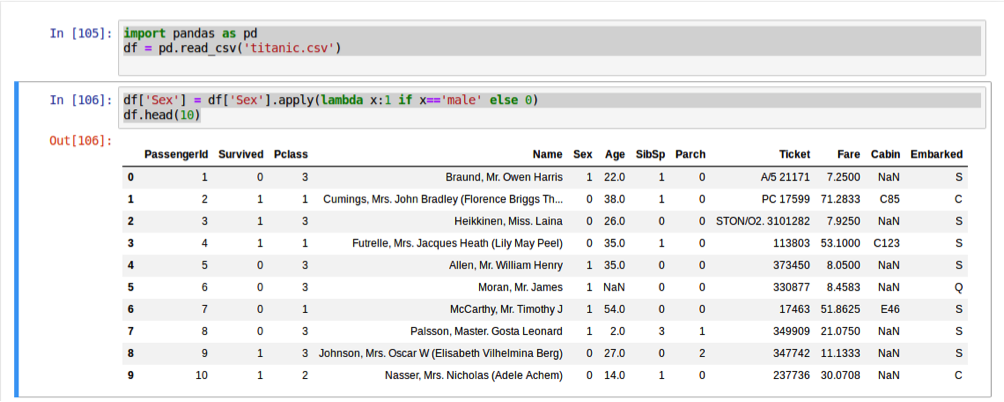Figure 12

Applying user-defined function on Data Set:

The functionality that we have done by using the if condition we can also perform it by making a user-defined function and then apply to all of the elements of data set:

`import pandas as pd`
`df = pd.read_csv('titanic.csv')`

`def a(x):`
`    if x=='male':`
`        return 1`
`    else:`
`        return 0`

`df['Sex'] = df['Sex'].apply(lambda x: a(x))`
`df.head(10)`

This will give the same result as generated by the program using the IF condition.

However, why we have to use this? What is the reason for doing this when we can also make a looping function? The reason is that these functions are much faster than in conventional ways. This difference cannot be observed when data is small, but it has a significant impact when the data is enormous, this function makes it easy and fast to execute.

Get Description of Data:

To get the description of complete data before doing anything will be good pandas make it very easy. It gives an overall description of the data on what type of data is present. Description can be obtained by describe function.

Describe function tells the overall count of the data, mean, standard deviation, etc.

Following is the way to get the description of data: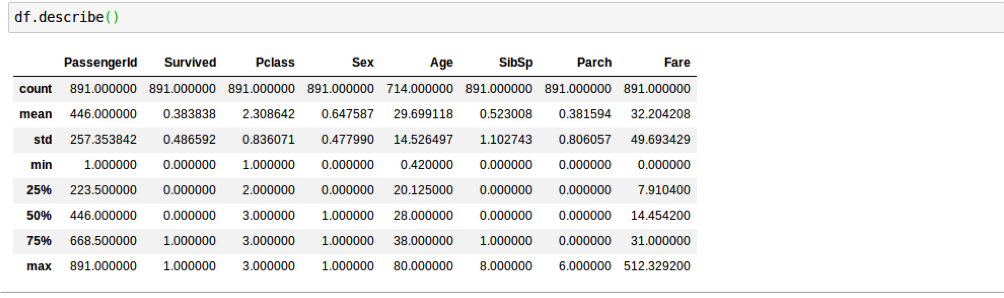Figure 13

This data is not so important when data is categorical but when data is continuous as in case of some company opening and closing stocks data of 5 years than this data will be handy to understand the data much more straightforward.

Null Elements:

We check the elements if there is any null element in pandas. We can count null elements and then decide whether we have to remove that null element, or we have to replace the value with some appropriate value. Following is the code to check that if there is any null value in data or not:

`import pandas as pd`
`df = pd.read_csv('titanic.csv')`
`df.isna().sum()`

The output that is generated is as follows:

 PassengerId      0 Survived         0 Pclass           0 Name             0 Sex              0 Age            177 SibSp            0 Parch            0 Ticket           0 Fare             0 Cabin          687 Embarked         2 dtype: int64

So here we can see there as 177 null age vale, and cabin data have 687 rows null. We can drop the null values and replace the value with some other value.

First, we will see how to drop the row:

`import pandas as pd`
`df = pd.read_csv('titanic.csv')`
`print (len(df))`

`df = df.dropna(axis=0)`
`print (df.isna().sum())`
`print (len(df))`

Now the output will be:

 Output 891   PassengerId    0 Survived       0 Pclass         0 Name           0 Sex            0 Age            0 SibSp          0 Parch          0 Ticket         0 Fare           0 Cabin          0 Embarked       0 dtype: int64   183

Now we can see the length of rows before dropping null value was 891 and after removing it the length of data frames become 183, which is bad we remove a major portion of data in such scenario we can replace the value:

`import pandas as pd`
`df = pd.read_csv('titanic.csv')`

`df = df.drop(['Cabin'],axis=1)`
`df = df.fillna(0)`

`df.isna().sum()`

we drop the column of the cabin because there are too many null values and then there only left age column to fill NA value in the data frame to zero after this the output will be as follows:

 PassengerId    0 Survived       0 Pclass         0 Name           0 Sex            0 Age            0 SibSp          0 Parch          0 Ticket         0 Fare           0 Embarked       0 dtype: int64

Now there are no null values. Notice we have mentioned axis parameter when dropping the column and dropping NA value the axis is referred to the rows and column. Axis 0 is referred to row, and axis one is referred to as a column. So, it is to tell the axis which a user wants to drop.

In the above example, we fill the NA values of all data column. To fill the NA value of the specific data column following method is to be applied:

`import pandas as pd`
`df = pd.read_csv('titanic.csv')`
`df = df.drop(['Cabin'],axis=1)`
`df['Age'] = df['Age'].fillna(0)`
`df.isna().sum()`